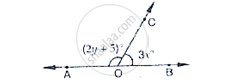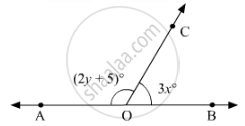# In the below Fig, OA and OB are opposite rays. If y = 35°, what is the value of x? - Mathematics

In the below Fig, OA and OB are opposite rays.

If y = 35°, what is the value of x?#### Solution 1

Given that if y = 35°

∠AOC + ∠BOC = 180°

(2 y + 5) + 3x = 180°

(2 (35) + 5) + 3x = 180°

(70 + 5) + 3x = 180°

3x = 180° - 75°

3x = 105°

x = 35°

x = 35°

#### Solution 2

In figure:

Since OA and OB are opposite rays. Therefore, AB is a line. Since, OC stands on line AB.

Thus,∠AOCand ∠BOC form a linear pair, therefore, their sum must be equal to180°.Or, we can say that

∠AOC + ∠BOC = 180°

From the given figure:

∠AOC= (2y + 5)and ∠BOC = 3x

On substituting these two values, we get

(2y + 5) + 3x = 180

3x + 2y = 180 -5

3x + 2y = 175                            ...(i)

On putting in y =35 in equation (A), we get:

3x + 2(35) =175

3x + 70 = 175

3x = 175 - 70

3x = 105

x = 105/3

x = 35

Hence, the value of x is 35.

Concept: Pairs of Angles
Is there an error in this question or solution?
Chapter 10: Lines and Angles - Exercise 10.2 [Page 14]

#### APPEARS IN

RD Sharma Mathematics for Class 9
Chapter 10 Lines and Angles
Exercise 10.2 | Q 1.2 | Page 14

Share